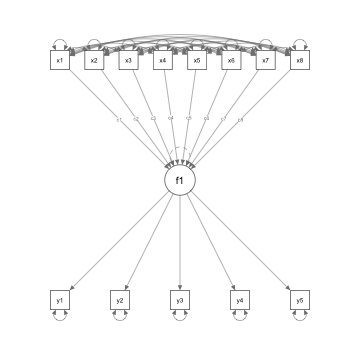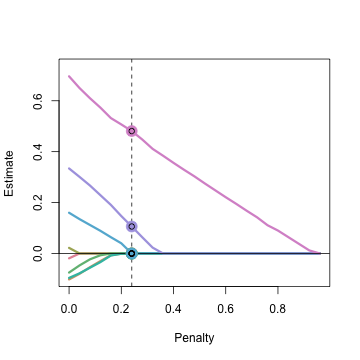# Regsem Package

### Simulate Data

To test out the regsem package, we will first simulate data

``````library(lavaan);library(regsem)
``````
``````## This is lavaan 0.6-15
## lavaan is FREE software! Please report any bugs.
``````
``````## Loading required package: Rcpp
``````
``````## Loading required package: Rsolnp
``````
``````sim.mod <- "
f1 =~ 1*y1 + 1*y2 + 1*y3+ 1*y4 + 1*y5
f1 ~ 0*x1 + 0*x2 + 0*x3 + 0*x4 + 0*x5 + 0.2*x6 + 0.5*x7 + 0.8*x8
f1~~1*f1"
dat.sim = simulateData(sim.mod,sample.nobs=100,seed=12)
``````

### Run the Model with Lavaan

And then run the model with lavaan so we can better see the structure.

``````run.mod <- "
f1 =~ NA*y1 + y2 + y3+ y4 + y5
f1 ~ c1*x1 + c2*x2 + c3*x3 + c4*x4 + c5*x5 + c6*x6 + c7*x7 + c8*x8
f1~~1*f1
"
lav.out <- sem(run.mod,dat.sim,fixed.x=FALSE)
#summary(lav.out)
parameterestimates(lav.out)[6:13,] # just look at regressions
``````
``````##    lhs op rhs label    est    se      z pvalue ci.lower ci.upper
## 6   f1  ~  x1    c1 -0.019 0.111 -0.172  0.863   -0.237    0.198
## 7   f1  ~  x2    c2 -0.102 0.111 -0.917  0.359   -0.320    0.116
## 8   f1  ~  x3    c3  0.022 0.113  0.193  0.847   -0.199    0.243
## 9   f1  ~  x4    c4 -0.075 0.122 -0.612  0.540   -0.313    0.164
## 10  f1  ~  x5    c5 -0.096 0.112 -0.857  0.391   -0.316    0.124
## 11  f1  ~  x6    c6  0.160 0.130  1.234  0.217   -0.094    0.415
## 12  f1  ~  x7    c7  0.334 0.148  2.263  0.024    0.045    0.624
## 13  f1  ~  x8    c8  0.696 0.135  5.163  0.000    0.432    0.960
``````

### Plot the Model

``````semPlot::semPaths(lav.out)
``````One of the difficult pieces in using the cv_regsem function is that the penalty has to be calibrated for each particular problem. In running this code, I first tested the default, but this was too small given that there were some parameters > 0.4. After plotting this initial run, I saw that some parameters weren’t penalized enough, therefore, I increased the penalty jump to 0.05 and with 30 different values this tested a model (at a high penalty) that had all estimates as zero. In some cases it isn’t necessary to test a sequence of penalties that would set “large” parameters to zero, as either the model could fail to converge then, or there is not uncertainty about those parameters inclusion.

``````reg.out <- cv_regsem(lav.out,n.lambda=30,type="lasso",jump=0.04,
pars_pen=c("c1","c2","c3","c4","c5","c6","c7","c8"))
``````

In specifying this model, we use the parameter labels to tell cv_regsem which of the parameters to penalize. Equivalently, we could have used the extractMatrices function to identify which parameters to penalize.

``````# not run
extractMatrices(lav.out)["A"]
``````

Additionally, we can specify which parameters are penalized by type: “regressions”, “loadings”, or both c(“regressions”,“loadings”). Note though that this penalizes all parameters of this type. If you desire to penalize a subset of parameters, use either the parameter name or number format for pars_pen.

Next, we can get a summary of the models tested.

``````summary(reg.out)
``````
``````## CV regsem Object
##  Number of parameters regularized: 8
##  Lambda ranging from 0 to 0.96
##  Lowest Fit Lambda: 0.24
##  Metric: BIC
##  Number Converged: 25
``````

## Plot the parameter trajectories

``````plot(reg.out)
``````Here we can see that we used a large enough penalty to set all parameter estimates to zero. However, the best fitting model came early on, when only a couple parameters were zero.

regsem defaults to using the BIC to choose a final model. This shows up in the final_pars object as well as the lines in the plot. This can be changed with the metric argument.

Understand better what went on with the fit

``````head(reg.out\$fits,10)
``````
``````##       lambda conv   rmsea      BIC    chisq
##  [1,]   0.00    0 0.06069 3959.819 50.49164
##  [2,]   0.04    0 0.05553 3951.021 50.90404
##  [3,]   0.08    0 0.05785 3952.039 51.92170
##  [4,]   0.12    0 0.06130 3953.624 53.50699
##  [5,]   0.16    0 0.06304 3951.247 55.73492
##  [6,]   0.20    0 0.06675 3953.155 57.64243
##  [7,]   0.24    0 0.06331 3941.757 60.06064
##  [8,]   0.28    0 0.06684 3943.714 62.01715
##  [9,]   0.32    0 0.07332 3950.653 64.35092
## [10,]   0.36    0 0.07119 3943.165 66.07319
``````

One thing to check is the “conv” column. This refers to convergence, with 0 meaning the model converged.

And see what the best fitting parameter estimates are.

``````reg.out\$final_pars[1:13] # don't show variances/covariances
``````
``````## f1 -> y1 f1 -> y2 f1 -> y3 f1 -> y4 f1 -> y5 x1 -> f1 x2 -> f1 x3 -> f1
##    1.065    0.949    1.033    1.119    1.039    0.000    0.000    0.000
## x4 -> f1 x5 -> f1 x6 -> f1 x7 -> f1 x8 -> f1
##    0.000   -0.001    0.000    0.106    0.481
``````

In this final model, we set the regression paths for x2,x3, x4, and x5 to zero. We make a mistake for x1, but we also correctly identify x6, x7, and x8 as true paths .Maximum likelihood estimation with lavaan had p-values > 0.05 for the parameters simulated as zero, but also did not identify the true path of 0.2 as significant (< 0.05).